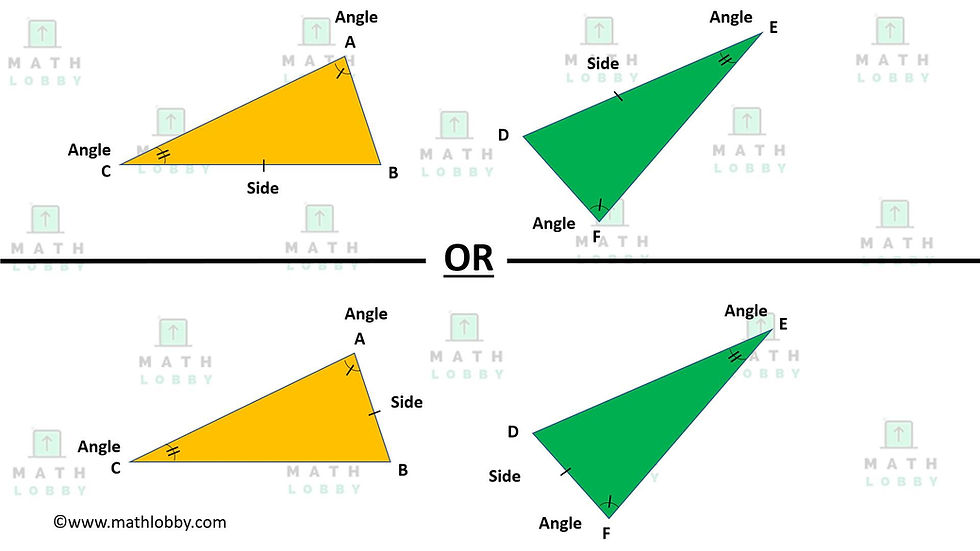top of page
Search

# Congruent Triangles

Updated: Nov 22, 2020

Dear Secondary Math students, Math Lobby will be covering the topic Congruent Triangles today.

In this note, you will learn:

1) What are congruent triangles?

2) Ways to prove that two triangles are congruent (SSS, SAS, ASA, AAS, RHS)

### 1) What are congruent triangles?

By definition, two triangles are said to be congruent if they have the corresponding sides that are of the same length, or the corresponding interior angles that are measured to be the same. The triangles must be of same shape and size, but not in the same orientation. When triangles are congruent, we use the sign “≅” to represent the relations between the triangles.

Let’s take a look at an example below:As shown in the diagram above, it can be seen that the corresponding sides of the triangles are of the same length (ie. AC = FE, CB = ED, BA = DF), and likewise for the interior angles, they are all measured to be the same (ie. ∡ABC = ∡FDE, ∡BCA = ∡DEF, ∡CAB = ∡EFD). Hence, we can say that triangle ABC is congruent to triangle FDE, or ΔABC ≅ ΔFDE.

*Note: Whenever we are proving triangles to be congruent, we must always remember to mention the corresponding vertexes in the correct order. For example, triangle ABC is congruent to triangle FDE, but not DEF or EFD because the vertex of A does not correspond with the vertex D or E.

### Ways to prove that two triangles are congruent (SSS, SAS, ASA, AAS, RHS)

However, it is not necessary for two triangles to fulfill all conditions to prove that they are congruent with each other. In fact, only three conditions have to be met in order to prove their congruency with one another. There are a few ways to go about this:

### 1) Side-Side-Side (SSS)

When both triangles have all three corresponding sides are equal in length### 2) Side-Angle-Side (SAS)

When both triangles have a pair of corresponding sides and the included angle are equal

*Note: An “included angle” means that it is the angle included between two sides of a triangle, which in this case, is the angle in between the corresponding sides of both the triangles.### 3) Angle-Side-Angle (ASA)

When both triangles have a pair of corresponding angles and the included side are equal

*Note: Likewise, for the definition of “included side”, which is the side included between two angles of a triangle, which in this case, is the side in between the corresponding angles of both the triangles.### 4) Angle-Angle-Side (AAS)

When both triangles have a pair of corresponding angles and a non-included side are equal### 5) Right-angle-Hypotenuse-Side (RHS)

This last method of proof is a little different, because it is only applicable for right-angled triangles. Both the triangles must have a right-angle, a corresponding hypotenuse and side of equal length.Why does Angle-Angle-Angle (AAA) or Side-Side-Angle (SSA) not work?

Angle-Angle-Angle (AAA) does not work because even if the two triangles have different sizes, they could still have the same three angles, which hence does not fulfill the requirement of the triangles being the same sizes. These are, instead, called similar triangles.Side-Side-Angle (SSA) does not work as well because there are other possible orientations to the triangles that satisfy the values, hence it is insufficient to prove the congruency of the triangles.And that’s all we have for today, students! Math Lobby hopes that after reading this article, you have a clear understanding of the definition regarding congruent triangles, the numerous ways to prove the congruency of two triangles and the answers to common questions asked by many.

As always: Work hard, stay motivated and we wish all students a successful and enjoyable journey with Math Lobby!

If you want to receive daily Secondary Math Tips,

FOLLOW our Instagram page at https://www.instagram.com/mathlobbymotivation/